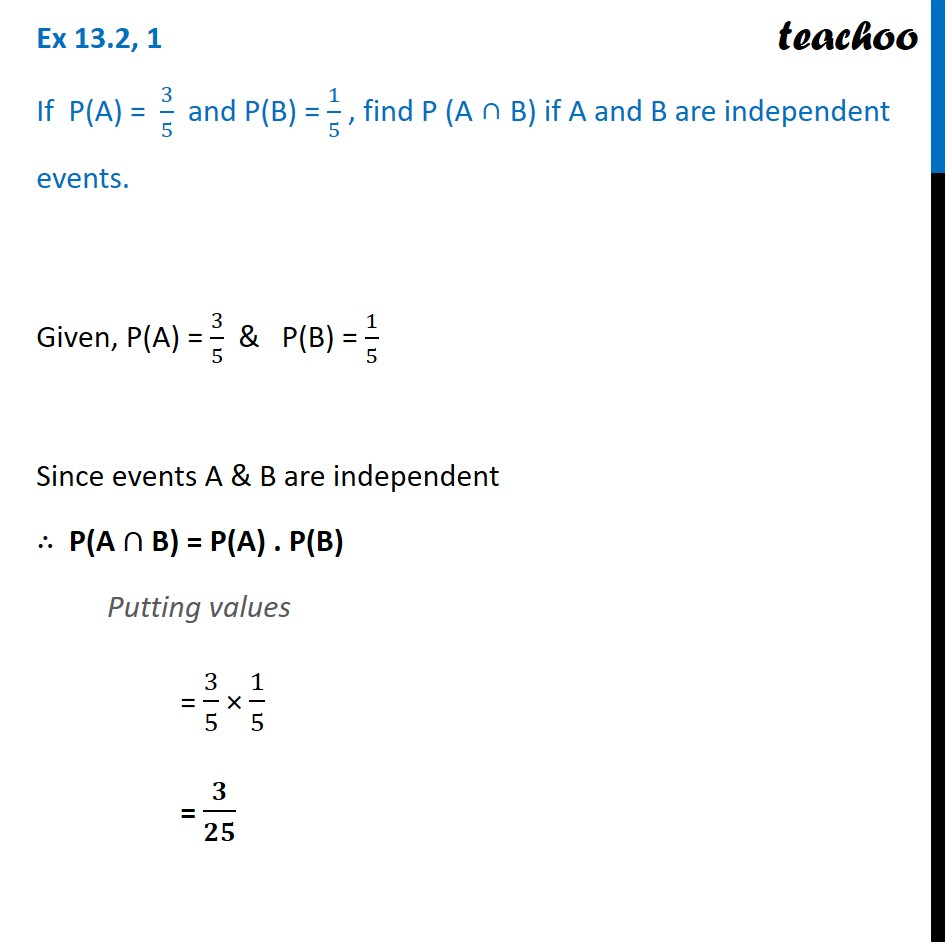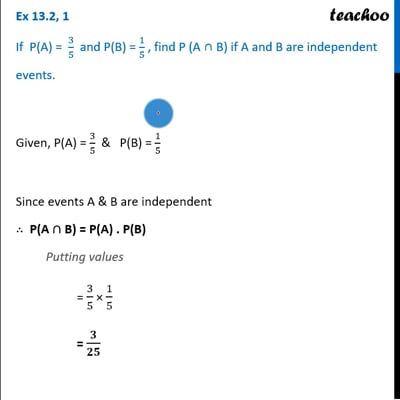Ex 13.2

Chapter 13 Class 12 Probability
Serial order wiseThis video is only available for Teachoo black users

Solve all your doubts with Teachoo Black (new monthly pack available now!)

### Transcript

Ex 13.2, 1 If P(A) = 3/5 and P(B) = 1/5 , find P (A ∩ B) if A and B are independent events.Given, P(A) = 3/5 & P(B) = 1/5 Since events A & B are independent ∴ P(A ∩ B) = P(A) . P(B) Putting values = 3/5 × 1/5 = 𝟑/𝟐𝟓